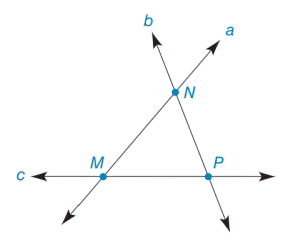Chapter 7.2, Problem 2EElementary Geometry For College St...

7th Edition
Alexander + 2 others
ISBN: 9781337614085

Solutions

Chapter
SectionElementary Geometry For College St...

7th Edition
Alexander + 2 others
ISBN: 9781337614085
Textbook Problem

Note: Exercises preceded by an asterisk are of a more challenging nature.a) In the figure, are lines a, b, and c concurrent?b) If one exists, name the point of concurrence for lines a, b, and c.To determine

(a)

To find:

In the figure, are lines a, b, and c concurrent.

Explanation

Definition:

Concurrent:

A number of lines are concurrent if they have exactly one point in common.

Two or more lines in a plane that have a single point in common are known as concurrent lines. When exactly two lines have a point in common, we generally describe them as intersecting lines rather than concurrent lines.

Given:

To determine

(b)

To find:

If one exists, name the point of concurrence for lines a, b, and c.

Still sussing out bartleby?

Check out a sample textbook solution.

See a sample solution

The Solution to Your Study Problems

Bartleby provides explanations to thousands of textbook problems written by our experts, many with advanced degrees!

Get Started

Expand each expression in Exercises 122. (y3+2y2+y)(y2+2y1)

Finite Mathematics and Applied Calculus (MindTap Course List)

[(13)2]3

Applied Calculus for the Managerial, Life, and Social Sciences: A Brief Approach

Factoring Completely Factor the expression completely. 99. x2 2x 8

Precalculus: Mathematics for Calculus (Standalone Book)

Can the vectors in Problems 1-4 be probability vectors? If not, why? 3.

Mathematical Applications for the Management, Life, and Social Sciences

For y = sin2 x + cos2 x, y = _____. a) 2 sin x 2 cos x b) 2 sin x cos x c) 4 sin x cos x d) 0

Study Guide for Stewart's Single Variable Calculus: Early Transcendentals, 8th